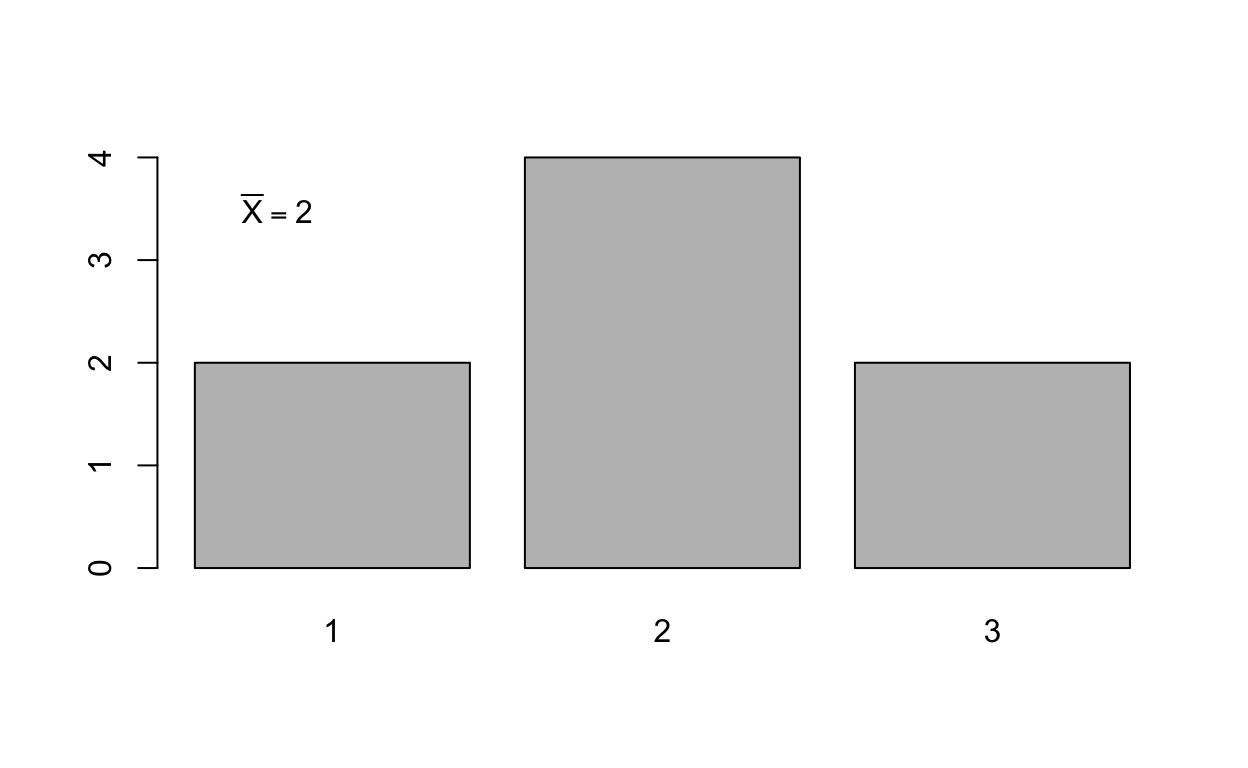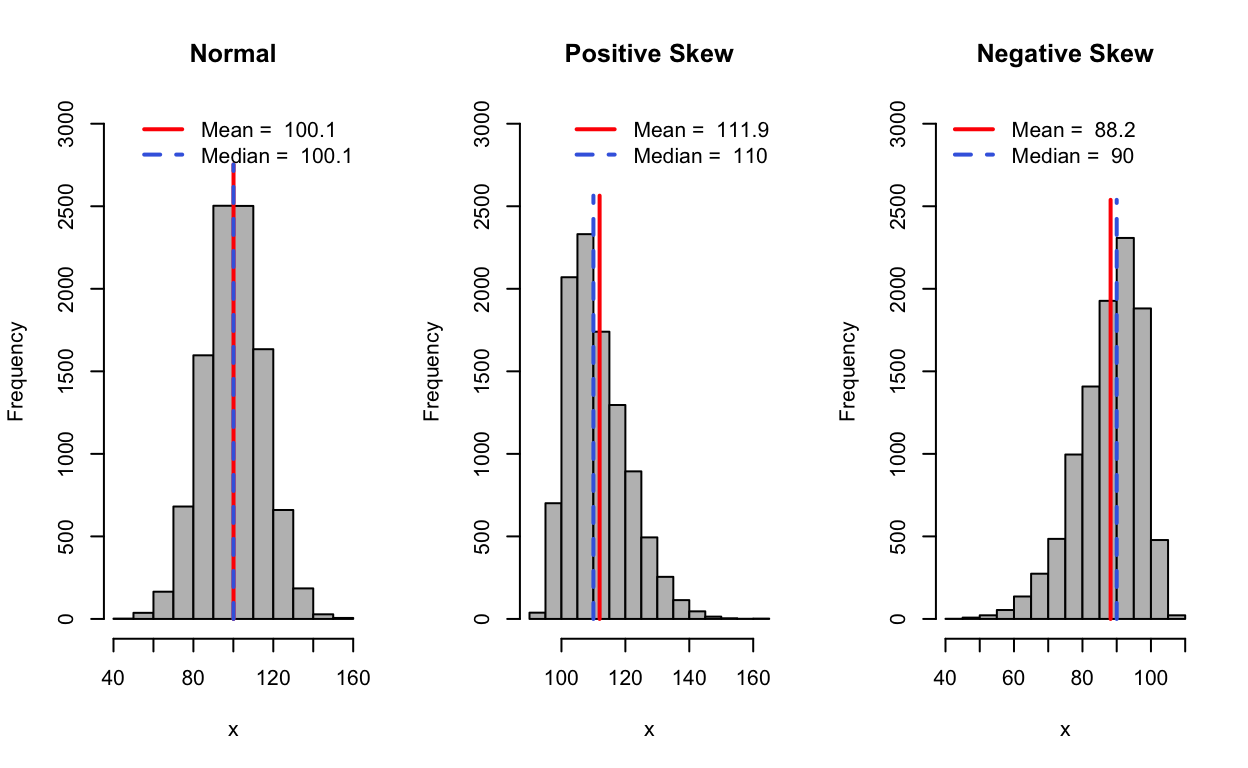# Measures of Central Tendency

Central Tendency is an important statistics in much of research. The goal is to simplify our data to one representative value. Before you do this you must make sure your measure of central tendency is appropriate for your data. In this tutorial I will introduce you to the most common ways to calculate central tendency

William Murrah www.statistical-thinking.com (QMER)aub.ie/qmer
2020-09-16

### Packages used in this tutorial

library(sn)
library(mosaic)

source("code/ct_hist.R")


## Notation

Scientific notation, while often confusing and frustrating initially, is very useful in helping to convey complex ideas in a compact and precise manner. The table below contains scientific notations relevant to this tutorial on central tendency and the next on variability.

We will be using the following notation in this class:

## Important Notation

Symbol meaning
$$y$$ Dependent Variable
$$x$$ Independent Variable
$$N$$ Population size
$$n$$ Sample size
$$\Sigma$$ Sum of
$$\mu$$ Population mean
$$M$$ or $$\bar{X}$$ Sample mean
$$M_w$$ or $$\bar{X}_w$$ Weighted mean

## Mean

### Calculating the mean

$\text{Population mean: } \mu = \frac{\Sigma x}{N}$

$\text{Sample mean: } \bar{x} = \frac{\Sigma x}{n}$

$$x$$ = (1, 1, 2, 2, 2, 2, 3, 3)

$$n$$ = 8

### Calculate sample mean:

$\bar{x} = \frac{\Sigma x}{n} = \frac{1+1+2+2+2+2+3+3}{8} = \frac{16}{8} = 2$

# Create a vector of numbers named scores
scores <- c(1, 1, 2, 2, 2, 2, 3, 3)
# Calculate the mean 'by hand'
sum(scores)/length(scores)

 2
# Calculate the mean using mean()
mean(scores)

 2

### Means summarize a distribution

We can table scores to see the frequency distribution,

table(scores)

scores
1 2 3
2 4 2 

which shows we have two scores that have the value 2, four scores that have the value 2, and two scores that have the value 3.

We can plot the frequency distribution as a bar plot.

barplot(table(scores))
text(0.5, 3.5, expression(bar(X) == 2))Both the table and the plot suggest that a score of 2 represents a typical or central score in this distribution of scores.

### Characteristics of the Mean

1. Changing an existing score changes the mean
2. Adding a new score or removing an existing score will change the mean, unless the value of this score is equal to the mean.
3. Adding, subtracting, multiplying, or dividing each score in a distribution by a constant will cause the mean to change by that constant.
4. The sum of the differences of scores from their mean is zero.
5. The sum of the squared differences of scores from their mean is minimal.

This last point is particularly important, so I will demonstrate it with our scores variable.

c_scores <- scores - mean(scores) # Scores centered around the mean.
cbind(scores, c_scores)

     scores c_scores
[1,]      1       -1
[2,]      1       -1
[3,]      2        0
[4,]      2        0
[5,]      2        0
[6,]      2        0
[7,]      3        1
[8,]      3        1
sum(c_scores)

 0

Because the mean is like the center of mass of the data, the scores above the mean cancel out the scores below the mean.

## Median

The median is the middle score, with half of the scores falling above and half below this score. To calculate the median first order the values of the variable from smallest to largest. If n is odd, the median is the middle value in the sorted variable, or the value that is in the $$\frac{n + 1}{2}$$ position.

If the n is even, then the median value is the average of the middle two values of the sorted variable. So, for our example variable x

$$x$$ = (1, 1, 2, 2, 2, 2, 3, 3)

because $$n = 8$$, we average the middle two values, which are both $$2$$, so the median of $$x$$ is $$2$$.

median(scores)

 2

## Mode

The mode is the most frequent value observed in a variable. NOTE: The mode() function in R does NOT calculate this form of central tendency, but does something very different. So don’t use that function to get the most common value in a variable. Instead you can use the table() function.

table(scores)

scores
1 2 3
2 4 2 

## Choosing a measure of central tendency

When appropriate, the mean is the preferred measure of central tendency for most applications. This is because we can do the most with the mean mathematically. Recall from what we learned about the characteristics of the mean that we can meaningfully add, subtract, multiply and divide means. But this depends on the type of variable we are dealing with. Generally speaking, the mean is useful for numeric variables that are symmetric in shape, preferably normally distributed. When data are skewed (i.e. not symmetrical), the mean can be adversely impacted by the skewness, being pulled toward the skewed tail more so than the median. Therefore with skewed distributions the median can be a better measure of central tendency. The median is also less susceptible to the influence of outliers.

### An illustrative example

If three people who earn typical salaries are sitting at a bar and Bill Gates sits down beside them, the average salary at the bar just went up drastically, and doesn’t represent anyone there. But the median salary, would still be a good representation of most of the people at the bar.

The median U.S. salary is about $45,000. Bill Gates makes about$3,710,000,000, or 3.7 billion dollars a year. We can “simulate” this in R:

normal_salaries <- c(40000, 45000, 50000)
billGates_salary <- 3.71e9
# Salaries after Bill Gates sits down at the bar
awkward_conversation <- c(normal_salaries, billGates_salary)


Let’s look at the measures of central tendency before Bill Gates strolls into the bar.

mean(normal_salaries)

 45000
median(normal_salaries)

 45000

With no outliers, both our measures give us a good sense of the typical salary at the bar.

What happens when a billionaire sits down?

# After Bill Gates sits down
mean(awkward_conversation)

 927533750
median(awkward_conversation)

 47500

Now the mean salary is $927,533,750, which is way more than the three original patrons make, and much less than Bill Gates makes. So, the mean is not a good measure of central tendency here, but the median, which is$47,500 is still representative of the typical person at the bar.

## Skew and Central Tendency

Notice from the example above, that outliers tend to pull the mean toward the tail of the distribution with the extreme cases. We can see that in the plots below.

set.seed(1234)
n <- 10000
xbar <- 100
sigma <- 15
nrm <- round(rnorm(n, xbar, sigma),2)

psk <- round(rsn(n, xi = xbar, omega = sigma, alpha = 5))
nsk <- round(rsn(n, xi = xbar, omega = sigma, alpha = -5))

par(mfrow = c(1, 3))
ct_hist(nrm, main = "Normal", ylim = c(0, 3000))
ct_hist(psk, main = "Positive Skew", ylim = c(0, 3000))
ct_hist(nsk, pos = "topleft", main = "Negative Skew", ylim = c(0, 3000))par(mfrow = c(1,1))


When the distribution is symmetrical with no extreme values, the mean and median are typically good measures of central tendency, but with skew, the mean is pulled to the extreme tail more so than the median, and it is often beneficial to use the median. This is why you often hear about median income instead of mean income. You can use this to your advantage. Often we can get the mean and median of a distribution, and if they are substantially different, we can not only tell that there is skew, but we can tell what direction the skew is in. See if you can figure out how to do this from the graph above.